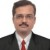# Modeling the continuous and discrete factors together

Six Sigma – iSixSigma Forums General Forums Methodology Modeling the continuous and discrete factors together

Viewing 6 posts - 1 through 6 (of 6 total)
• Author
Posts
• #53607Rupesh Lochan
Member

Hi,
I need to create a model for bringing predictability into the system. The challange is that the factors are a mix of discrete as well as continuous variables. Can someone suggest which method to use for modeling ?
I am not in a position to use DOE, since it will be quite time taking and experimenting with live data will be difficut…hence am constrained to work with the historical data.

Regards,

0
#190842broderick
Participant

How you analyze your data is not dependent upon what type of data you are using in terms of happenstance vs observational/experimental, rather it is the form in which you find your dependent and independent variables. For example, if your response variable is continuous and your predictors are some combination, you can run a linear regression model to get a decent predictive y. Or use logistical regression to model a respsonse variable that is ordinal, binary, or nominal. Or you could chose an entirely different class of model that provides a more useful model for your purposes. Good Luck.

0
#190843Rupesh Lochan
Member

Let me make myself more clear about the issue.
I have my Y continuous. I have Xs which have been found signifcant through different hypothesis testing techniquess. There are such 6 Xs in total. Three are continuous and rest three are discrete.
I am looking for an equation involving all 6 Xs for predicting my Y. Simple linear regression is certainly not applicable since it is an OFAT technique and can take care of only one continuous factor. Multiple regression can take care of more than one factors but all should be continuous.
Question is: which technique can be used for getting such equation invlving all continuous and dicrete factors together?

0
#190844Robert Butler
Participant

The statement “Multiple regression can take care of more than one factors but all should be continuous” is in error. You can use both continuous and categorical/discrete variables as X’s in multiple regression.

If the discrete variables are ordinal (i.e. Lickert Scale) then you can use them as you would any other X. If they are categorical and nominal then you will need to use dummy variables to represent their levels in the regression equation.

For example you have factories North, East, and West and you want to include factory as an X variable. Then you would set up dummy variables 1 and 2 as follows:

Factory DV1 DV2
North 0 0
East 1 0
West 0 1

and when you run the regression the variables you would include for factory would be DV1 and DV2. For additional information check any good book on regression methods and look in the index for “dummy variable”.

A much bigger issue as far as your historical data is concerned will be your ability to detect significant correlations with those variables known to be important to the process. This will be an issue because if they are known to be important they will most likely have been subject to control and thus there is a very good chance that they will have been controlled to the extent that their impact on the process will not result in a significant correlation with the Y values of interest.

In addition to the above there is the problem of variable confounding. Unless you have some very unusual historical data it is unlikely that your X’s of interest exhibit enough independence of each other to permit their inclusion in a meaningful regression equation. This doesn’t mean that the regression package won’t allow them in the equation (this would only happen if the confounding was perfect) but it will mean that any attempt on your part to attribute variation in the Y to changes in a particular X will likely fail when you attempt to verify the equation.

0
#190845Rupesh Lochan
Member

Very good inputs. Thanks Robert.
I was doing the same by coding the attribute variables as 1,2,3,.. etc. I have 2 questions:
1. What is different when the attribute variable data is ordinal? I will just like to code the levels of x as 1,2 3,4,5
2. Can’t I get the “Best Subset” out of all the variables ( continous + attribute ), which will take care of multicolinearity and confounding…. and then run regression to get the model ? Your thought ??
2. Have you used “General Regression” option of Minitab 16. I see the results are not the same as “Regression” option. Why it is so ?

0
#190846Robert Butler
Participant

If the attribute variable isn’t ordinal then your choice of 1,2,3 is completely arbitrary and you can induce patterns in the response where none exist. For example if we characterize the factories of North, East, and West as 1,2,3 and the measured response from these factories are respectively 5, 15, and 10 then when you run the regression you will find a curvilinear effect due to factory. If we change the code to 1,3,2 for North, East, and West, the curvilinear effect disappears and all we have is a simple linear trend. If the attribute is ordinal then direction is implied and you can run the analysis without having to use dummy variables.

Best subset methods will not take care of multicollinearity and confounding. In order to check for these things you will have to test your X matrix using VIF’s and condition indices from an eigenvalue analysis. It’s my understanding that Minitab has the VIF capability but cannot do an eigenvalue assessment.

I don’t know Minitab so I don’t know much about the options with respect to regression (I use SAS).

0
Viewing 6 posts - 1 through 6 (of 6 total)

You must be logged in to reply to this topic.